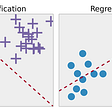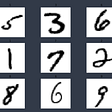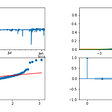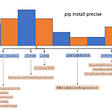# Reinforcement Learning for Stock Trading

1. action — the mechanism by which the agent transitions between states of the environment
2. agent — the entity that uses a policy to maximize expected return gained from transitioning between states of the environment.
3. environment — the world that contains the agent and allows the agent to observe that world’s state
4. reward — the numerical result of taking an action in a state, as defined by the environment
5. state — the parameter values that describe the current configuration of the environment, which the agent uses to choose an action

2. Sell
3. Hold

# Reward

`model = Sequential()        model.add(Dense(units=64, input_dim=self.state_size, activation="relu"))        model.add(Dense(units=32, activation="relu"))        model.add(Dense(units=8, activation="relu"))        model.add(Dense(self.action_size, activation="linear"))        model.compile(loss="mse", optimizer=Adam(lr=0.001))`
`def act(self, state):          if not self.is_eval and np.random.rand() <= self.epsilon:          return random.randrange(self.action_size)           options = self.model.predict(state)          return np.argmax(options)`

# Experience Replay

`def expReplay(self, batch_size):         mini_batch = []         l = len(self.memory)         for i in range(l - batch_size + 1, l):      mini_batch.append(self.memory[i])          for state, action, reward, next_state, done in mini_batch:        target = reward               if not done:                       target =reward+self.gamma*\            np.amax(self.model.predict(next_state)0])                           target_f = self.model.predict(state)                   target_f[action] = target                   self.model.fit(state, target_f, epochs=1, verbose=0)            if self.epsilon > self.epsilon_min:                   self.epsilon *= self.epsilon_decay`

`for e in range(episode_count + 1):        print("Episode " + str(e) + "/" + str(episode_count))        state = getState(data, 0, window_size + 1)         total_profit = 0        agent.inventory = []         for t in range(l):                action = agent.act(state)                         # hold        next_state = getState(data, t + 1, window_size + 1)                reward = 0          # buy        if action == 1:                        agent.inventory.append(data[t])                       print("Buy: " + formatPrice(data[t]))                         # sell        elif action == 2 and len(agent.inventory) > 0:                bought_price = agent.inventory.pop(0)                         reward = max(data[t] - bought_price, 0)                              total_profit += data[t] - bought_price                                           print("Sell: " + formatPrice(data[t]) + " | Profit: " +                                   formatPrice(data[t] - bought_price))                 done = True if t == l - 1 else False        agent.memory.append((state, action, reward, next_state,                                                                 done))                  state = next_state                 if done:                       print("--------------------------------")                           print("Total Profit: " + formatPrice(total_profit))                       print("--------------------------------")                 if len(agent.memory) > batch_size:                         agent.expReplay(batch_size)          if e % 10 == 0:                agent.model.save("models/model_ep" + str(e))`

## Evaluation

`for t in xrange(l):     action = agent.act(state)          # hold    next_state = getState(data, t + 1, window_size + 1)     reward = 0        # buy    if action == 1:         agent.inventory.append(data[t])         print("Buy: " + formatPrice(data[t]))          # sell    elif action == 2 and len(agent.inventory) > 0:         bought_price = agent.inventory.pop(0)         reward = max(data[t] - bought_price, 0)         total_profit += data[t] - bought_price         print("Sell: " + formatPrice(data[t]) + " | Profit: " +                                                  formatPrice(data[t] - bought_price))      done = True if t == l - 1 else False     agent.memory.append((state, action, reward, next_state, done))    state = next_state      if done:         print("--------------------------------")         print(stock_name + " Total Profit: "                                    +formatPrice(total_profit))       print("--------------------------------")`

# References

--

--

--

## More from Abhishek Maheshwarappa

Data Scientist | ML-Ops| https://abhi-gm.github.io/ | https://www.linkedin.com/in/abhishek-g-m/ | https://github.com/abhi-gm

Love podcasts or audiobooks? Learn on the go with our new app.

## How Transfer Learning works## Hyper-parameter Optimization with Manual Gradient — Part II: One-step Learning rate Optimization## Introduction to Machine Learning for beginners## Recognizing celebs in a music video using deep learning under 5-minutes## Batch and Streaming in the World of Data Science and Data Engineering## How No-Code-ML Tools Carry Out The Hardwork For Data Scientists?## Number Recognition with Python## Improve vision models by pretrain on uncurated images without supervision with SEER## Abhishek Maheshwarappa

Data Scientist | ML-Ops| https://abhi-gm.github.io/ | https://www.linkedin.com/in/abhishek-g-m/ | https://github.com/abhi-gm

## Crypto Market Forecast: StatsModels VARMAX Method## Measuring Hedge Fund Performance with Factor Model Monte Carlo## Forecasting Bitcoin Prices using ARMA, GARCH and Neural Networks## Optimizing a Portfolio of Models